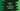# Python Set intersection_update() Method## Python set intersection_update() method explanation with example:

intersection_update method is defined in python set and this method can be used to remove all items those are not present in the given sets. This method updates the set directly. In this post, we will learn how to use intersection_update method with example.

### Definition of intersection_update:

intersection_update method is defined as below:

``set.intersection_update(first_set, second_set,....)``

We can pass any number of sets to this method.

### Parameters and return value:

It can take an arbitary number of arguments. It doesn’t return anything. It directly updates the caller set. This is the difference between intersection and intersection_update. It needs atleast one set.

It doesn’t return anything or it returns None.

### Example of intersection_update:

Let’s take a look at an example:

``````if __name__ == '__main__':
first_set = {'a', 'b', 'c', 'd'}
second_set = {'b', 'c', 'd'}

first_set.intersection_update(second_set)

print(first_set)``````

It will print the below output:

``{'c', 'b', 'd'}``

As you can see here, we are calling intersection_update on first_set and second_set is passed as the parameter to it. Since a is not in second_set, but it is in first_set, it is removed from first_set.

### Example with more than one parameter:

The above example uses only one set as the parameter. We can also pass more than one set as the parameters. Let’s take a look at the below program:

``````if __name__ == '__main__':
first_set = {'a', 'b', 'c', 'd'}
second_set = {'b', 'c'}
third_set = {'b'}

first_set.intersection_update(second_set, third_set)

print(first_set)``````

It will print b. Because that is the only common element among first_set, second_set and third_set.